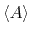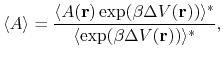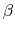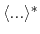Next: Gaussian Accelerated Molecular Dynamics Up: Accelerated Sampling Methods Previous: Accelerated Sampling Methods   Contents   Index

Subsections

## Accelerated Molecular Dynamics

Accelerated molecular dynamics (aMD)  is an enhanced-sampling method that improves the conformational space sampling by reducing energy barriers separating different states of a system. The method modifies the potential energy landscape by raising energy wells that are below a certain threshold level, while leaving those above this level unaffected. As a result, barriers separating adjacent energy basins are reduced, allowing the system to sample conformational space that cannot be easily accessed in a classical MD simulation.

Please include the following two references in your work using the NAMD implementation of aMD:

• Accelerated Molecular Dynamics: A Promising and Efficient Simulation Method for Biomolecules, D.Hamelberg, J.Mongan, and J.A. McCammon. J. Chem. Phys., 120:11919-11929, 2004.
• Implementation of Accelerated Molecular Dynamics in NAMD, Y.Wang, C.Harrison, K.Schulten, and J.A. McCammon, Comp. Sci. Discov., 4:015002, 2011.

### Theoretical background

In the original form of aMD , when the system's potential energy falls below a threshold energy,, a boost potential is added, such that the modified potential,, is related to the original potential,, via(82)

whereis the boost potential,(83)

As shown in the following figure, the threshold energycontrols the portion of the potential surface affected by the boost, while the acceleration factordetermines the shape of the modified potential. Note thatcannot be set to zero, otherwise the derivative of the modified potential is discontinuous.From an aMD simulation, the ensemble average,, of an observable,, can be calculated using the following reweighting procedure:(84)

in which=, andandrepresent the ensemble average in the original and the aMD ensembles, respectively.

Currently, aMD can be applied in three modes in NAMD: aMDd, aMDT, and aMDdual . The boost energy is applied to the dihedral potential in the aMDd mode (the default mode), and to the total potential in the aMDT mode. In the dual boost mode (aMDdual) , two independent boost energies are applied, one on the dihedral potential and the other on the (Total - Dihedral) potential.

### NAMD parameters

The following parameters are used to enable accelerated MD:

• accelMDIs accelerated molecular dynamics active?Acceptable Values: on or off
Default Value: off
Description: Specifies if accelerated MD is active.

• accelMDdiheApply boost to dihedrals?Acceptable Values: on or off
Default Value: on
Description: Only applies boost to the dihedral potential. By default, accelMDdihe is turned on and the boost energy is applied to the dihedral potential of the simulated system. When accelMDdihe is turned off, aMD switches to the accelMDT mode, and the boost is applied to the total potential.

• accelMDEThreshold energyAcceptable Values: Real number
Description: Specifies the threshold energyin the aMD equations.

• accelMDalphaAcceleration factorAcceptable Values: Positive real number
Description: Specifies the acceleration factorin the aMD equations.

• accelMDdualUse dual boost mode?Acceptable Values: on or off
Default Value: off
Description: When accelMDdual is on, aMD switches to the dual boost mode. Two independent boost potentials will be applied: one to the dihedral potential that is controlled by the parameters accelMDE and accelMDalpha, and a second to the (Total - Dihedral) potential that is controlled by the accelMDTE and accelMDTalpha parameters described below.

• accelMDTEThreshold energyin the dual boost modeAcceptable Values: Real number
Description: Specifies the threshold energyused in the calculation of boost energy for the (Total - Dihedral) potential. This option is only available when accelMDdual is turned on.

• accelMDTalphaAcceleration factorin the dual boost modeAcceptable Values: Positive real number
Description: Specifies the acceleration factorused in the calculation of boost energy for the (Total - Dihedral) potential. This option is only available when accelMDdual is turned on.

• accelMDFirstStepFirst accelerated MD stepAcceptable Values: Zero or positive integer
Default Value: 0
Description: Accelerated MD will only be performed when the current step is equal to or higher than accelMDFirstStep, and equal to or lower than accelMDLastStep. Otherwise regular MD will be performed.
• accelMDLastStepLast accelerated MD stepAcceptable Values: Zero or positive integer
Default Value: 0
Description: Accelerated MD will only be performed when the current step is equal to or higher than accelMDFirstStep, and equal to or lower than accelMDLastStep. Otherwise regular MD will be performed. Note that the accelMDLastStep parameter only has an effect when it is positive. When accelMDLastStep is set to zero (the default), aMD is `open-ended' and will be performed till the end of the simulation.

• accelMDOutFreqFrequency in steps of aMD outputAcceptable Values: Positive integer
Default Value: 1
Description: An aMD output line will be printed to the log file at the frequency specified by accelMDOutFreq. The aMD output will contain the boost potential () at the current timestep, the average boost potential () since the last aMD output, and various potential energy values at the current timestep. The boost potentialcan be used to reconstruct the ensemble average described earlier.Next: Gaussian Accelerated Molecular Dynamics Up: Accelerated Sampling Methods Previous: Accelerated Sampling Methods   Contents   Index
http://www.ks.uiuc.edu/Research/namd/